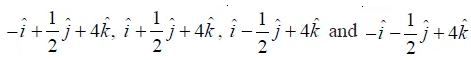NCERT Solutions for Maths Class 12 Exercise 10.4

# NCERT Solutions for Maths Class 12 Exercise 10.4

## NCERT Solutions for Maths Class 12 Exercise 10.4

Hello Students. Welcome to maths-formula.com. In this post, you will find the complete NCERT Solutions for Maths Class 12 Exercise 10.4.

You can download the PDF of NCERT Books Maths Chapter 10 for your easy reference while studying NCERT Solutions for Maths Class 12 Exercise 10.4.

Class 12th is a very crucial stage of your student’s life, since you take all important decisions about your career on this stage. Mathematics plays a vital role to take decision for your career because if you are good in mathematics, you can choose engineering and technology field as your career.

NCERT Solutions for Maths Class 12 Exercise 10.4 helps you to solve each and every problem with step by step explanation which makes you strong in mathematics.

All the schools affiliated with CBSE, follow the NCERT books for all subjects. You can check your syllabus from NCERT Syllabus for Mathematics Class 12.

NCERT Solutions for Maths Class 12 Exercise 10.4 are prepared by the experienced teachers of CBSE board. If you are preparing for JEE Mains and NEET level exams, then it will definitely make your foundation strong.

If you want to recall All Maths Formulas for Class 12, you can find it by clicking this link.

If you want to recall All Maths Formulas for Class 11, you can find it by clicking this link.

NCERT Solutions for Maths Class 12 Exercise 10.1

## NCERT Solutions for Maths Class 12 Exercise 10.4

Maths Class 12 Ex 10.4 Question 1.

Find |a × b|, if a = i – 7j + 7k and b = 3i – 2j + 2k.

Solution:

We have given that, a = i – 7j + 7k and b = 3i – 2j + 2k

Maths Class 12 Ex 10.4 Question 2.

Find a unit vector perpendicular to each of the vector a + b and ab, where a = 3i + 2j + 2k and b = i + 2j – 2k.

Solution:

We have given that, a = 3i + 2j + 2k and b = i + 2j – 2k
Therefore, a + b = (3i + 2j + 2k) + (i + 2j – 2k) = 4i + 4j

And ab = (3i + 2j + 2k) – (i + 2j – 2k) = i + 4k

Maths Class 12 Ex 10.4 Question 3.

If a unit vector a makes angles π/3 with i, π/4 with j and an acute angle θ with k, then find θ and hence the components of a.

Solution:

Let a = a1i + a2j + a3k such that a = 1

Maths Class 12 Ex 10.4 Question 4.

Show that (ab) × (a + b) = 2(a × b)

Solution:

Maths Class 12 Ex 10.4 Question 5.

Solution:

Maths Class 12 Ex 10.4 Question 6.

Given that a.b = 0 and a × b = 0. What can you conclude about the vectors a and b?

Solution:

Maths Class 12 Ex 10.4 Question 7.

Let the vectors a, b, c be given as a1i + a2j + a3k, b1i + b2j + b3k, c1i + c2j + c3k. Then show that a × (b + c) = a × b + a × c

Solution:

Maths Class 12 Ex 10.4 Question 8.

If either a = 0 or b = 0, then a × b = 0. Is the converse true? Justify your answer with an example.

Solution:

Maths Class 12 Ex 10.4 Question 9.

Find the area of the triangle with vertices A(1, 1, 2), B(2, 3, 5) and C(1, 5, 5).

Solution:

Maths Class 12 Ex 10.4 Question 10.

Find the area of the parallelogram whose adjacent sides are determined by the vectors a = ij + 3k and b = 2i – 7j + k.

Solution:

Maths Class 12 Ex 10.4 Question 11.

Let the vectors a and b be such that |a| = 3 and |b| = √2/3, then a × b is a unit vector, if the angle between a and b is
(A) π/6               (B) π/4         (C) π/3        (D) π/2

Solution:

We have given that

Hence, the correct answer is option (B).

Maths Class 12 Ex 10.4 Question 12.

Area of a rectangle having vertices A, B, C and D with position vectors, respectively is

(A) ½             (B) 1                 (C) 2                 (D) 4

Solution:

Hence, the correct answer is option (C).